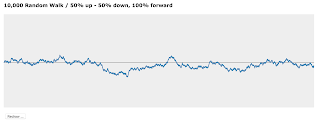## Wednesday 2 March 2016

### Random walk on graph and collapse of Bayesian Network

Random Walk
Consider the following Bayesian Network or Markov Chain, which shows your walking direction. Every time you want to take a step; the network says you have the equal chance of walking towards the north (n) or south (s).Two states Bayesian Network with equal probabilities for any state change

I've prepared simple graph based on d3.js for 10,000 steps; you can see it by clicking on the following link. The horizontal axis could be considered as time and the vertical one as the direction to the north or the south. There are equal probabilities to take a step toward the north or the south for the given link. Try the redraw button to see what happens each time it redraws it.

10,000 Random Walk / 50% up - 50% down, 100% forward ...A sample result of the 50/50 random walk.

Now if you just increase the north probability by 1% the state diagram would be like the following most of the time:Two states Bayesian Network with 51/49 probabilities for any state change

The following link shows a live version of such a walk. This time, whenever you want to take a step you have 51% chance of walking towards the north and 49% towards the south. (Note that this is not a walk with constant drift.)

10,000 Random Walk / 51% up - 49% down, 100% forward ...A sample result of the 51/49 random walk.

If you try to redraw this graph, you'll find the effect of 1% increase or decrease in the edges of the graph. With this introduction, I would like to continue talking about how we can predict the future based on a Bayesian Network.

Walking on Bayesian Network
The state diagrams we had in this post were nothing but simple Bayesian Networks; we can use the same idea to walk on more complicated Networks. A network which any of its nodes is the one of the many states of a system could also be considered as a Markov Chain. So walking from one node to another node is nothing but changing the system's states. You may need to review the following posts to have a better idea of what we are saying.

Using probability to predict state of a system
Introduction to Transition matrix and Superposition of states
More on Transition matrix and Superposition of states

We saw that to define the next state of a system we need to derive a transition matrix from the system's Bayesian Network or Markov Chain  and then use the following formula, in which T is the transition matrix and S(t) is the state vector of the system at time t:

S(t+1) = T . S(t)     

When we calculate the formula  to define the next state of the system, it gives us a vector with probabilities of being in any possible states. So for example for the above two simple networks, the result is a two-dimensional vector with corresponding probabilities for being in state "n" or "s" or P(n) and P(s). Now what happens when we want to take a step forward is like tossing a not necessarily fair coin with probability ratio of 50/50 or 51/49 for north/south and decide the next direction.

Moreover, for a system with more than two states, choosing the next step is like tossing an n-sided not fair dice with probability ratios for sides S1/S2/S3/... at time t equals to P(S1 @t)/P(S2 @t)/P(S2 @t)/... and deciding the next state. We can show the next step as the superposition of the possible states as we talked about it before in the above posts. However, when we toss the dice and take the next step the result of the equation  collapses and only one of the possible state stays, it is like your network eventually transforms to only path.

An Intuitive Example
Consider you are coming back from home and based on the previous experiment you have not more than these four options with the following probabilities:Options you have to do when you get home

Now the question is what would you do? You might answer it depends on the state you have, so if you do not feel hungry, definitely won't go for dinner. Alternatively, if you are not tired won't go for a rest or taking a shower, and so on and so forth.

That is correct your decision making always depends on the state you are. Now, what if we know all the answers to this questions or in another word we can precisely define your current situation, and the above graph shows the result of different options you may choose at this situation. Now, which one of them are you going to choose?

You have to be realistic the model had been made from a huge data gathering and specifies all the possible choices you could have in such a situation. You do not have any more information to make a decision. Again which path do you choose? You choose them randomly.

But not a from a truly random process. It is like a biased random or as we called it before, unfair random. You need to toss an unfair four-sided dice with probability ratios of 0.25/0.167/0.5/0.083 and see what happen. That is how the world works:

At the lowest layer of decision making where you do not have any more precise information which defines your current state, this is a random walk on a graph which selects one of the options.

I am going to write another post under the name of "People do sin, no matter who they are!" and describe how a Bayesian Network or Markov Chain could model any decision making.